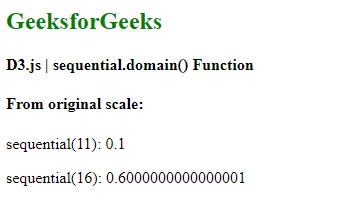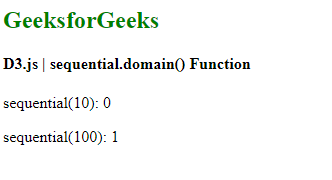Skip to content
Related Articles
D3.js sequential.domain() Function
• Last Updated : 31 Aug, 2020

Sequential scales are very similar to continuous scale. On a continuous scale, mapping is done in a continuous way between domain and range. The only difference is that the output range of this scale is fixed by its interpolator and this range cannot be changed.

The sequential.domain() function is used to set the domain of the given sequential scale. The domain array always accepts two numeric values.

Syntax:

`sequential.domain([domain]);`

Parameters: This function accepts a single parameter as mentioned above and described below:

• domain: This sets the minimum and maximum domain value for the scale.

Return Values: This function does not return any value.

Example 1:

## HTML

 ` ``<``html` `lang``=``"en"``> `` ` `<``head``> ``    ``<``meta` `charset``=``"UTF-8"` `/> ``    ``<``meta` `name``=``"viewport"``        ``path1tent="``width``=``device``-width, ``        ``initial-scale``=``1``.0"/> `` ` `    ``<``script` `src``=``        ``"https://d3js.org/d3.v6.min.js"``>``    ```` `` ` `<``body``> ``    ``<``h2` `style``=``"color:green"``> GeeksforGeeks ``   ` `    ``<``h4``> D3.js | sequential.domain() Function ``   ` `    ``<``script``> ``        ``var sequential = d3.scaleSequential()``            ``// Setting domain to [10,20]``            ``.domain([10,20]);`` ` `        ``document.write("<``h4``>From original scale:");`` ` `        ``document.write("<``p``>sequential(11): ", ``                    ``sequential(11) + "");`` ` `        ``document.write("<``p``>sequential(16): ", ``                    ``sequential(16) + "");``    `` ```` ` ``

Output:Example 2: When a domain is given as string numbers it is parsed to number.

## HTML

 ` ``<``html` `lang``=``"en"``> `` ` `<``head``> ``    ``<``meta` `charset``=``"UTF-8"` `/> ``    ``<``meta` `name``=``"viewport"``        ``path1tent="``width``=``device``-width, ``        ``initial-scale``=``1``.0"/> `` ` `    ``<``script` `src``=``        ``"https://d3js.org/d3.v6.min.js"``>``    ```` `` ` `<``body``> ``    ``<``h2` `style``=``"color:green"``> GeeksforGeeks ``   ` `    ``<``h4``> D3.js | sequential.domain() Function ``   ` `    ``<``script``> ``        ``var sequential = d3.scaleSequential()``            ``// Setting domain to ["10","100"]``            ``.domain(["10","100"]);`` ` `        ``document.write("<``p``>sequential(10): ", ``                    ``sequential(10) + "");`` ` `        ``document.write("<``p``>sequential(100): ", ``                    ``sequential(100) + "");``    `` ```` ` ``

Output:My Personal Notes arrow_drop_up